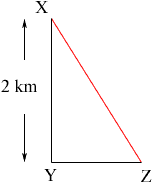Quandaries and Queries Who is asking: Student Level: Middle Question: X is due north of Y and 2km distant. Z is due east of Y and has a bearing of S35°12'E from X. How far, to the nearest metre, is Z from X? Hi, The diagram I drew is below.Since X is due north of Y and Z is due east of Y, the angle XYZ is a right angle. Since Z has a bearing of S35°12'E from X, the measure of the angle ZXY is 35o 12'. (There is a good explanation of bearings at a page kept by David Levesen, a faculty member in the Department of Geology at Brooklyn College, City Uiversity of New York.) Hence  2/|XZ| = cos(35o 12') Penny Go to Math Central